The K5 Learning Blog urges parents to be pro-active in helping their children reach their full academic potential.

K5 Learning
provides free worksheets, workbooks and an online reading and math program for kindergarten to grade 5 students.# How to convert decimals to fractions

Decimals can be expressed as a fraction. Converting decimals to fractions is usually a two-step process:

1.    Depending on the number of decimal places, convert the fraction using tenths, hundredths, thousandths, etc.
2.    Simplify the fraction to the lowest common term.

Let’s demonstrate this with an example:

The decimal is 0.4.

## Convert the fraction

Write down 0.4 divided by 1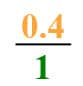Now, multiply both top and bottom by 10 for every number after the decimal point.

In our example, there is one number after the decimal point, so multiply by 10.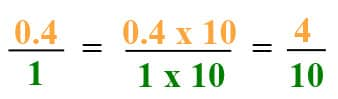This chart is a more visual way to show decimals as common fractions.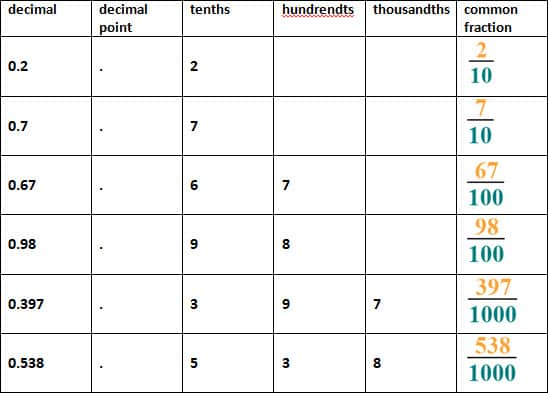## Simplify the fraction

Let’s move onto the second part: simplifying the fraction.

This is best demonstrated with an example.
The decimal is 0.64. Now we need to convert it into a fraction.The common factors of 64 and 100 are 1, 2 and 4
The greatest common factor is 4
Divide both numbers with 4
64 ÷ 4 = 16
100 ÷ 4 = 25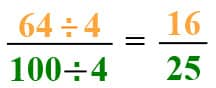The fraction in its simplest form is: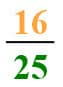In our grade 4 math section, we have free worksheets to practice converting decimals to fractions.